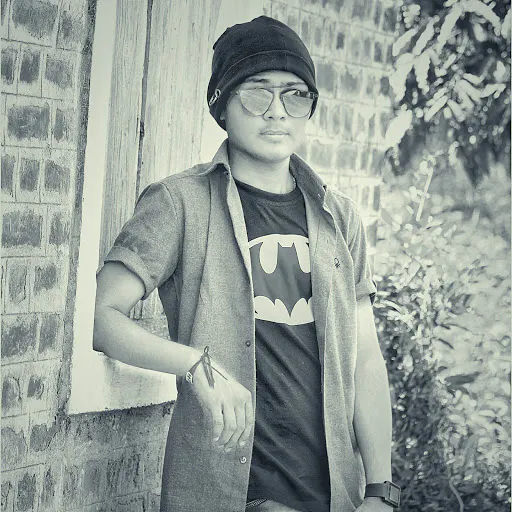Published

# Distance Measurement Using Ultrasonic Sensor

Distance measurement using an ultrasonic sensor.

IntermediateProtip1,502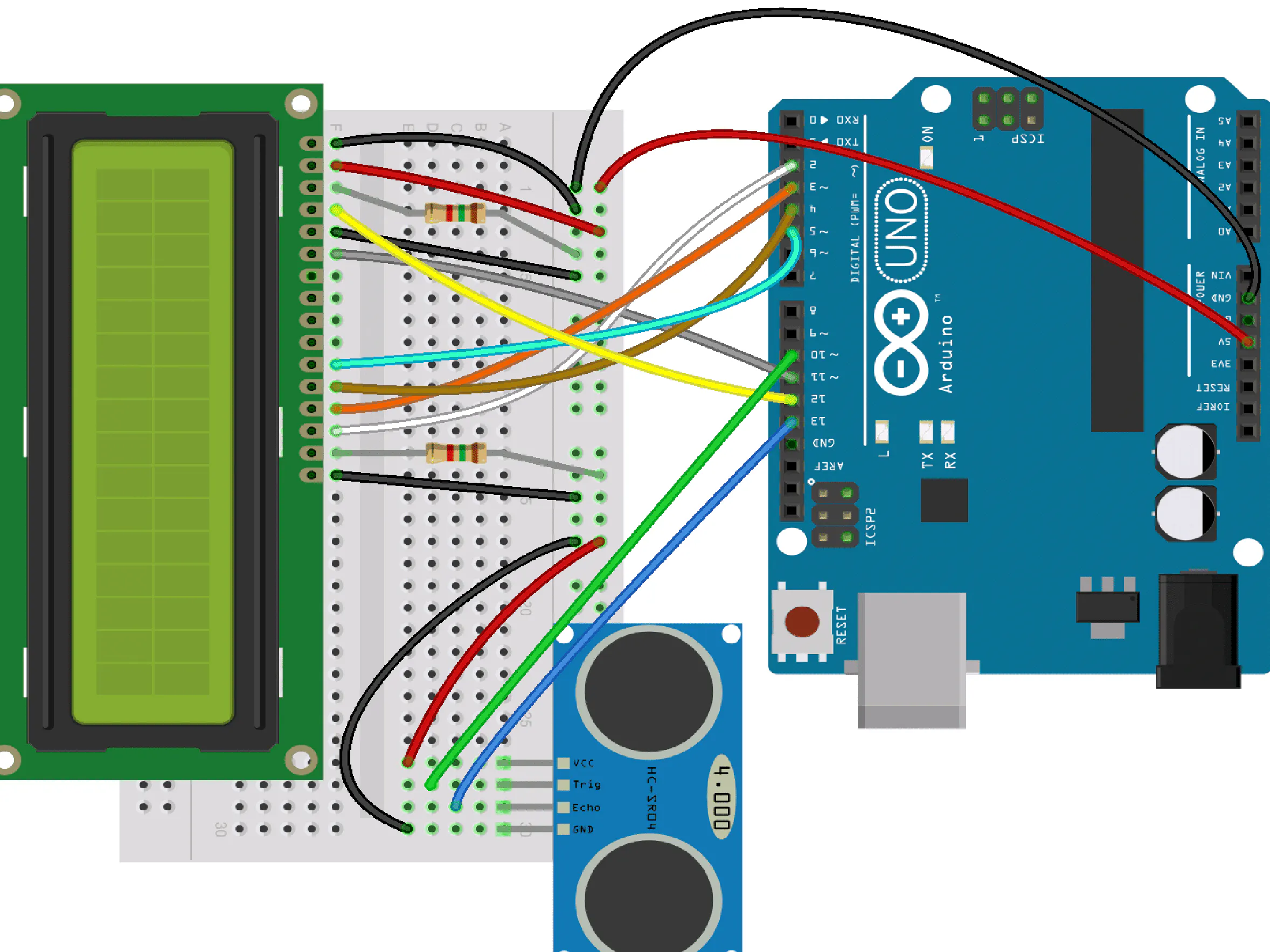## Things used in this project

### Hardware componentsArduino UNO & Genuino UNO
×1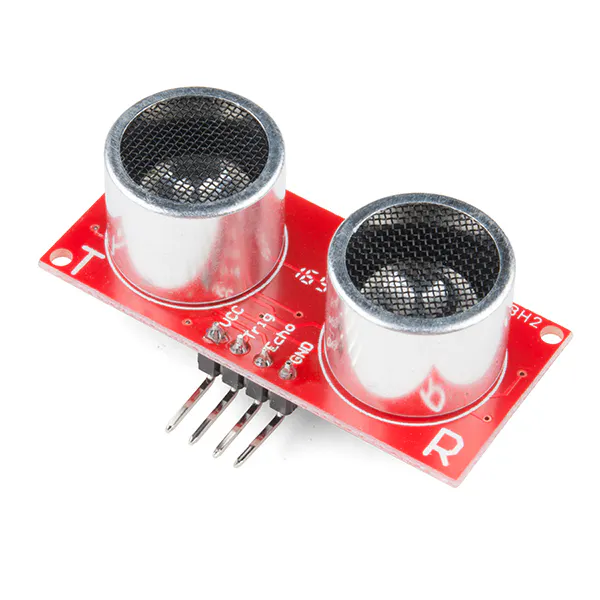SparkFun Ultrasonic Sensor - HC-SR04
×1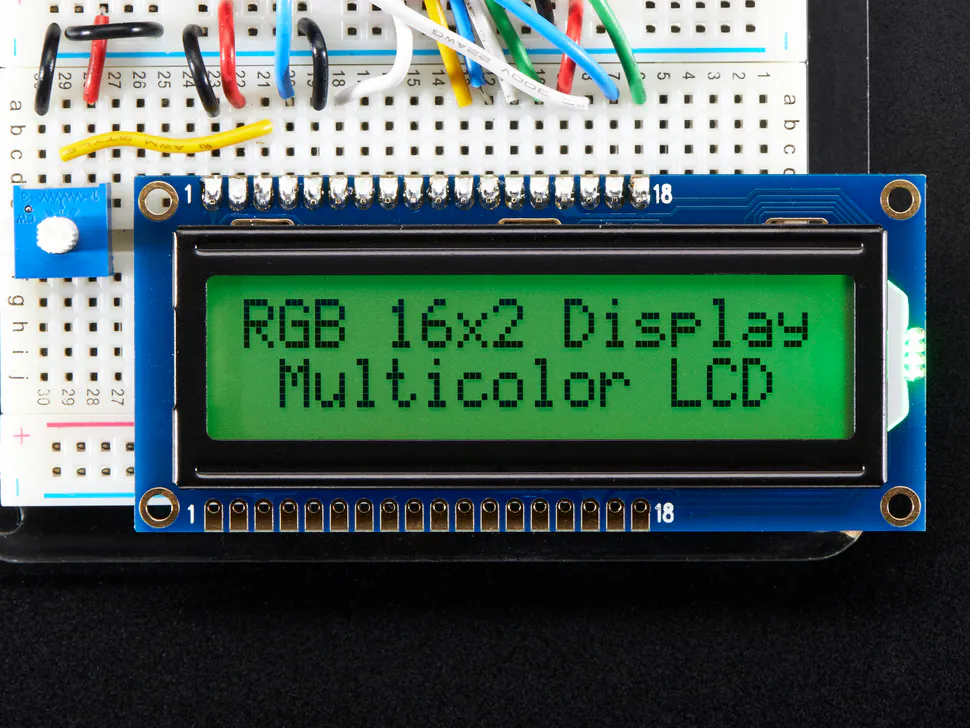Adafruit RGB Backlight LCD - 16x2 diplay distance
×1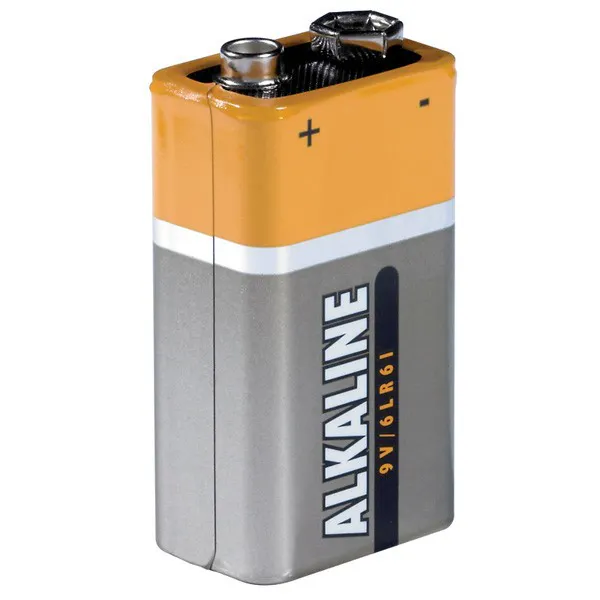9V battery (generic)
×1

## Code

### arduino code

Arduino
```#include<LiquidCrystal.h>
const int rs=12, en=3, d4=4, d5=5, d6=6, d7=7 ;
const int trig=2,echo=9;
int distanc;
long d;
LiquidCrystal lcd(rs,en,d4,d5,d6,d7);

void setup() {

lcd.begin(16,2);
pinMode(trig,OUTPUT);
pinMode(echo,INPUT);
Serial.begin(9600);
}

void loop() {

digitalWrite(trig,LOW);
delay(2);
digitalWrite(trig,HIGH);
delay(10);
digitalWrite(trig,LOW);
d=pulseIn(echo,HIGH);
distanc= d*0.034/2;
lcd.setCursor(0,0);
lcd.print("distance");
lcd.setCursor(0,1);
lcd.print(distanc);

}
```

## Credits

### Smit Babariya

10 projects • 9 followers